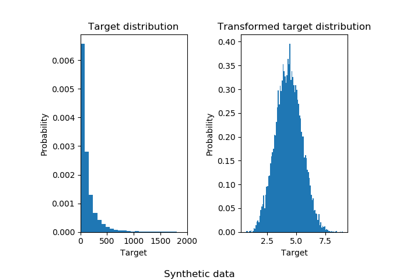# sklearn.compose.TransformedTargetRegressor¶

class sklearn.compose.TransformedTargetRegressor(regressor=None, transformer=None, func=None, inverse_func=None, check_inverse=True)[source]

Meta-estimator to regress on a transformed target.

Useful for applying a non-linear transformation in regression problems. This transformation can be given as a Transformer such as the QuantileTransformer or as a function and its inverse such as log and exp.

The computation during fit is:

regressor.fit(X, func(y))


or:

regressor.fit(X, transformer.transform(y))


The computation during predict is:

inverse_func(regressor.predict(X))


or:

transformer.inverse_transform(regressor.predict(X))


Read more in the User Guide.

Parameters: regressor : object, default=LinearRegression() Regressor object such as derived from RegressorMixin. This regressor will automatically be cloned each time prior to fitting. transformer : object, default=None Estimator object such as derived from TransformerMixin. Cannot be set at the same time as func and inverse_func. If transformer is None as well as func and inverse_func, the transformer will be an identity transformer. Note that the transformer will be cloned during fitting. Also, the transformer is restricting y to be a numpy array. func : function, optional Function to apply to y before passing to fit. Cannot be set at the same time as transformer. The function needs to return a 2-dimensional array. If func is None, the function used will be the identity function. inverse_func : function, optional Function to apply to the prediction of the regressor. Cannot be set at the same time as transformer as well. The function needs to return a 2-dimensional array. The inverse function is used to return predictions to the same space of the original training labels. check_inverse : bool, default=True Whether to check that transform followed by inverse_transform or func followed by inverse_func leads to the original targets. regressor_ : object Fitted regressor. transformer_ : object Transformer used in fit and predict.

Notes

Internally, the target y is always converted into a 2-dimensional array to be used by scikit-learn transformers. At the time of prediction, the output will be reshaped to a have the same number of dimensions as y.

Examples

>>> import numpy as np
>>> from sklearn.linear_model import LinearRegression
>>> from sklearn.compose import TransformedTargetRegressor
>>> tt = TransformedTargetRegressor(regressor=LinearRegression(),
...                                 func=np.log, inverse_func=np.exp)
>>> X = np.arange(4).reshape(-1, 1)
>>> y = np.exp(2 * X).ravel()
>>> tt.fit(X, y)
TransformedTargetRegressor(...)
>>> tt.score(X, y)
1.0
>>> tt.regressor_.coef_
array([2.])


Methods

 fit(self, X, y[, sample_weight]) Fit the model according to the given training data. get_params(self[, deep]) Get parameters for this estimator. predict(self, X) Predict using the base regressor, applying inverse. score(self, X, y[, sample_weight]) Returns the coefficient of determination R^2 of the prediction. set_params(self, \*\*params) Set the parameters of this estimator.
__init__(self, regressor=None, transformer=None, func=None, inverse_func=None, check_inverse=True)[source]
fit(self, X, y, sample_weight=None)[source]

Fit the model according to the given training data.

Parameters: X : {array-like, sparse matrix}, shape (n_samples, n_features) Training vector, where n_samples is the number of samples and n_features is the number of features. y : array-like, shape (n_samples,) Target values. sample_weight : array-like, shape (n_samples,) optional Array of weights that are assigned to individual samples. If not provided, then each sample is given unit weight. self : object
get_params(self, deep=True)[source]

Get parameters for this estimator.

Parameters: deep : boolean, optional If True, will return the parameters for this estimator and contained subobjects that are estimators. params : mapping of string to any Parameter names mapped to their values.
predict(self, X)[source]

Predict using the base regressor, applying inverse.

The regressor is used to predict and the inverse_func or inverse_transform is applied before returning the prediction.

Parameters: X : {array-like, sparse matrix}, shape = (n_samples, n_features) Samples. y_hat : array, shape = (n_samples,) Predicted values.
score(self, X, y, sample_weight=None)[source]

Returns the coefficient of determination R^2 of the prediction.

The coefficient R^2 is defined as (1 - u/v), where u is the residual sum of squares ((y_true - y_pred) ** 2).sum() and v is the total sum of squares ((y_true - y_true.mean()) ** 2).sum(). The best possible score is 1.0 and it can be negative (because the model can be arbitrarily worse). A constant model that always predicts the expected value of y, disregarding the input features, would get a R^2 score of 0.0.

Parameters: X : array-like, shape = (n_samples, n_features) Test samples. For some estimators this may be a precomputed kernel matrix instead, shape = (n_samples, n_samples_fitted], where n_samples_fitted is the number of samples used in the fitting for the estimator. y : array-like, shape = (n_samples) or (n_samples, n_outputs) True values for X. sample_weight : array-like, shape = [n_samples], optional Sample weights. score : float R^2 of self.predict(X) wrt. y.

Notes

The R2 score used when calling score on a regressor will use multioutput='uniform_average' from version 0.23 to keep consistent with metrics.r2_score. This will influence the score method of all the multioutput regressors (except for multioutput.MultiOutputRegressor). To specify the default value manually and avoid the warning, please either call metrics.r2_score directly or make a custom scorer with metrics.make_scorer (the built-in scorer 'r2' uses multioutput='uniform_average').

set_params(self, **params)[source]

Set the parameters of this estimator.

The method works on simple estimators as well as on nested objects (such as pipelines). The latter have parameters of the form <component>__<parameter> so that it’s possible to update each component of a nested object.

Returns: self

## Examples using sklearn.compose.TransformedTargetRegressor¶Effect of transforming the targets in regression model